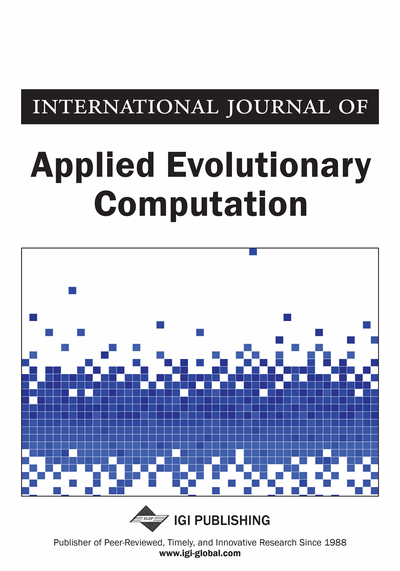# Ant Custering Algorithms

Yu-Chiun Chiou, Shih-Ta Chou
DOI: 10.4018/jaec.2010010101
OnDemand:
(Individual Articles)
Available
\$29.50
No Current Special Offers

## Abstract

This paper proposes three ant clustering algorithms (ACAs): ACA-1, ACA-2 and ACA-3. The core logic of the proposed ACAs is to modify the ant colony metaheuristic by reformulating the clustering problem into a network problem. For a clustering problem of N objects and K clusters, a fully connected network of N nodes is formed with link costs, representing the dissimilarity of any two nodes it connects. K ants are then to collect their own nodes according to the link costs and following the pheromone trail laid by previous ants. The proposed three ACAs have been validated on a small-scale problem solved by a total enumeration method. The solution effectiveness at different problem scales consistently shows that ACA-2 outperforms among these three ACAs. A further comparison of ACA-2 with other commonly used clustering methods, including agglomerative hierarchy clustering algorithm (AHCA), K-means algorithm (KMA) and genetic clustering algorithm (GCA), shows that ACA-2 significantly outperforms them in solution effectiveness for the most of cases and also performs considerably better in solution stability as the problem scales or the number of clusters gets larger.
Article Preview
Top

## 1. Introduction

Clustering, so-called set partitioning, is a basic methodology widely applied in the fields including statistics, mathematical programming (e.g., location selecting, network partitioning, routing, scheduling and assignment problems) and computer science (e.g., pattern recognition, learning theory, image processing and computer graphics). The core logic of clustering is to classify all objects into several mutually exclusive groups so as to achieve some optimal conditions. Most conventional clustering methods rapidly become computationally intractable as the problem scale gets larger due to the combinatorial nature of the methods. The mathematical model of clustering for a given number (K) of clusters can be formulated as:

[CA](1) (3)for all i, j(4)for all i, j(5) where xij denotes the relationship among objects. xij=1 indicates that object i is assigned to object j, (also cluster j). xij=0 otherwise; i, j={1,2,…,N}, N is the number of objects, K is the number of clusters. Accordingly, xjj=1 represents that object j is assigned to itself, indicating that cluster j has been formed. F(X) is the objective function. X is the vector of xij for all i, j. Equation (2) represents that each object must be assigned to one and only one object (cluster). Equation (3) represents that a total of exactly K clusters will be formed. Equation (4) represents that the object i can only be assigned to the object which is also assigned to itself, i.e. the corresponding cluster has been formed.

The total number of feasible solutions of [CA] is:. That is, the solution space of [CA] is exponential to the problem size and number of clusters. For instance, there are a total of 511 feasible solutions for 10 objects (N=10) to be divided into 2 clusters (K=2). There are a total of 42,525 feasible solutions for 10 objects to be divided into 5 clusters. Brucker (1978) and Welch (1983) proved that, for specific objective functions, clustering becomes an NP-hard problem when the number of clusters exceeds three, if one aims to find the optimal clusters. Hansen and Jaumard (1987) pointed out that even though the best algorithms developed for some specific objective functions, there would exhibit complexities of O(N3logN) or O(N3), leaving some room for further improvement.

## Complete Article List

Search this Journal:
Reset
Volume 14: 1 Issue (2023): Forthcoming, Available for Pre-Order
Volume 13: 4 Issues (2022): 2 Released, 2 Forthcoming
Volume 12: 4 Issues (2021)
Volume 11: 4 Issues (2020)
Volume 10: 4 Issues (2019)
Volume 9: 4 Issues (2018)
Volume 8: 4 Issues (2017)
Volume 7: 4 Issues (2016)
Volume 6: 4 Issues (2015)
Volume 5: 4 Issues (2014)
Volume 4: 4 Issues (2013)
Volume 3: 4 Issues (2012)
Volume 2: 4 Issues (2011)
Volume 1: 4 Issues (2010)
View Complete Journal Contents Listing